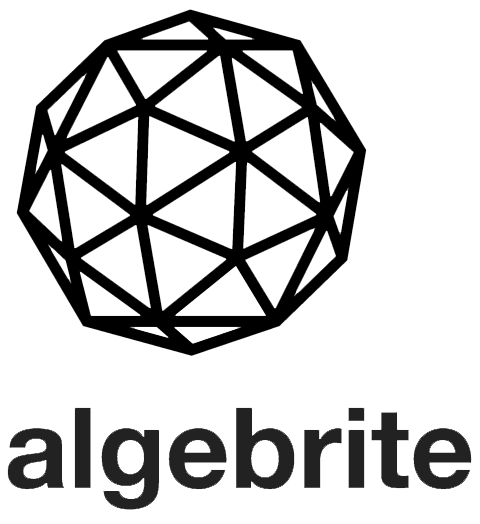# Algebrite 2016

Software

Algebrite is a Computer Algebra System in javascript. In fact Algebrite is currently the most advanced javascript computer algebra library.

Computer Algebra Systems (CAS) enable symbolic computation (in addition to numerical computation), allowing for example determination of exact solutions to problems rather than approximate ones, and even provide/support demonstration of properties of mathematical structures (see “matrices” example in animation below). They have many applications in the sciences and industry.

Algebrite supports: arbitrary-precision arithmetic, complex quantities, simplification, expansion , substitution, symbolic and numeric roots (i.e. solves equations), units of measurement, matrices, derivatives and gradients, tensors, integrals and multi-integrals (both finding primitives and computing them), and much more.

Algebrite is currently my most popular open source project, with over 460 stars on Github.

Examples (for new and more examples, check the website):